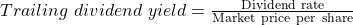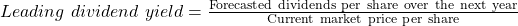Essential Concept 54: Commonly Used Price Multiples | IFT World
101 Concepts for the Level I Exam

# Essential Concept 54: Commonly Used Price MultiplesPrice to earnings (P/E): Ratio of a stock’s market price to its earnings per share.

There are two variations of P/E: the trailing P/E and the forward P/E.

• Trailing P/E is a stock’s current market price divided by the most recent four quarters’ EPS.
• The forward P/E or leading P/E, is a stock’s current price divided by next year’s expected earnings.

Rationales for using P/E include:

• Earnings are the key driver of value.
• P/E ratio is widely recognized and used by investors.
• Differences in P/Es may be related to differences in long-run average returns.

Drawbacks of using P/E include:

• Earnings can be negative which will make the ratio meaningless.
• Earnings often contain volatile, transient components.
• Management’s choice of accounting policies may taint EPS’s value.

Price to book value (P/B): Price divided by book value per share.

Book value per share represents, on a per-share basis, the investment that common shareholders have made in the company.  Book value will generally need to be adjusted.  When comparing, we often use tangible book value.

Rationales for using P/B include:

• Can be used when EPS is negative
• BVPS is more stable than EPS (especially when EPS is volatile)
• More useful for valuing companies with liquid assets (banks, etc.)
• Can be used when company is not a going concern
• Differences in P/B may relate to differences in long-run returns

Drawbacks of using P/B include:

• Some assets creating value (e.g., human capital) are not on the balance sheet
• Can be misleading when companies have different level of assets
• Accounting effects (e.g., R&D) can compromise BV
• BV is generally not reflective of market value

Price to sales (P/S): Ratio of market price per share to sales per share.

Rationale for using P/S include:

• Sales are less subject to accounting manipulation than EPS or BVPS
• Sales are positive even when EPS is negative
• Sales are more stable than EPS
• More useful in valuing mature, cyclical and zero income companies
• Differences in P/S may relate to differences in long-run returns

Drawbacks of using P/S include:

• A company may show sales growth despite low earnings and cash flow from operations.
• P/S does not capture differences in cost structures
• Does not capture different capital structures.
• Room for manipulating revenues remains

Price to cash flow (P/CF): Ratio of market price to cash flow per share.

Rationales for using P/CF:

• Cash flow is less prone to manipulation than earnings.
• More stable than EPS
• Addresses the issue of accounting differences
• Differences in P/CF may relate to differences in long-run returns

Drawbacks of using P/CF

• When cash flow is defined as earnings plus noncash charges, items such as noncash revenue and net changes in working capital are ignored.
• FCFE is viewed as more appropriate than cash flow. But, FCFE can be more volatile or negative for certain businesses.

Dividend yield: Ratio of dividend rate to market price per share.

The formulas for trailing and leading dividend yield are given below:where dividend rate = annualized amount of the most recent dividend

If the dividend is paid quarterly, then dividend rate = 4 * the most recent quarterly per-share dividend.Rationales for using dividend yield:

• Dividend yield is a component of total return.
• Dividends are less risky than capital appreciation in total return.

Drawbacks of using dividend yield:

• Since dividend yield is only a part of total return, not using all the parts make this valuation approach suboptimal.
• Dividend displacement of earnings is when investors choose to receive dividends now over future earnings growth.
• The assumption that dividends are less risky than the capital appreciation component implies that market prices are biased in the assessment of relative risk of the two components.